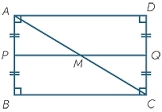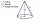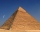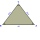# Area of a rectangle

Calculate the area of a rectangle with a diagonal of u = 12.5cm and a width of b = 3.5cm. Use the Pythagorean theorem.

Correct result:

S =  42 cm2

#### Solution:We would be pleased if you find an error in the word problem, spelling mistakes, or inaccuracies and send it to us. Thank you!Tips to related online calculators
Pythagorean theorem is the base for the right triangle calculator.

#### You need to know the following knowledge to solve this word math problem:

We encourage you to watch this tutorial video on this math problem:

## Next similar math problems:

• The base 2The base diameter of a right cone is 16cm and it's slant height is 12cm. A. ) Find the perpendicular height of the cone to 1 decimal place. B. ) Find the volume of the cone, convert to 3 significant figure. Take pie =3.14
• Perpendicular projectionDetermine the distance of a point B[1, -3] from the perpendicular projection of a point A[3, -2] on a straight line 2 x + y + 1 = 0.How long is a ladder that touches on a wall 4 meters high and its lower part is 3 meters away from the wall?
• Railway embankmentThe section of the railway embankment is an isosceles trapezoid, the sizes of the bases of which are in the ratio 5: 3. The arms have a length of 5 m and the height of the embankment is 4.8 m. Calculates the size of the embankment section area.
• The pyramidThe pyramid with a square base is 50 m high and the height of the sidewall is 80 m. Find the endge of the base of the pyramid.
• Isosceles triangleCalculate the area of an isosceles triangle, the base of which measures 16 cm and the arms 10 cm.
• Right triangleA right triangle ABC is given, c is a hypotenuse. Find the length of the sides a, b, the angle beta if c = 5 and angle alfa = A = 35 degrees.
• Isosceles triangleIn an isosceles triangle ABC with base AB; A [3,4]; B [1,6] and the vertex C lies on the line 5x - 6y - 16 = 0. Calculate the coordinates of vertex C.
• Find the 13Find the equation of the circle inscribed in the rhombus ABCD where A[1, -2], B[8, -3] and C[9, 4].
• Calculate 6Calculate the distance of a point A[0, 2] from a line passing through points B[9, 5] and C[1, -1].
• Integer sidesA right triangle with an integer length of two sides has one leg √11 long. How much is its longest side?
• Trip with compassDuring the trip, Peter went 5 km straight north from the cottage, then 12 km west and finally returned straight to the cottage. How many kilometers did Peter cover during the whole trip?
• Right angleIn a right triangle ABC with a right angle at the apex C, we know the side length AB = 24 cm and the angle at the vertex B = 71°. Calculate the length of the legs of the triangle.
• SailingSolve the following problem graphically. The fishing boat left the harbor early in the morning and set out to the north. After 12 km of sailing, she changed course and continued 9 km west. Then When she docked and reached the fishing grounds she launched
• Right triangle - ratioThe lengths of the legs of the right triangle ABC are in ratio b = 2: 3. The hypotenuse is 10 cm long. Calculate the lengths of the legs of that triangle.
• The right triangleIn the right triangle ABC with right angle at C we know the side lengths AC = 9 cm and BC = 7 cm. Calculate the length of the remaining side of the triangle and the size of all angles.
• Isosceles triangleCalculate the size of the interior angles and the length of the base of the isosceles triangle if the length of the arm is 17 cm and the height to the base is 12 cm.